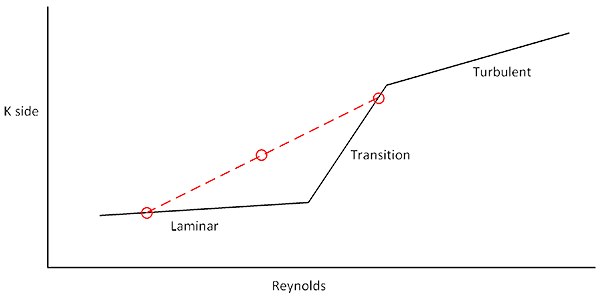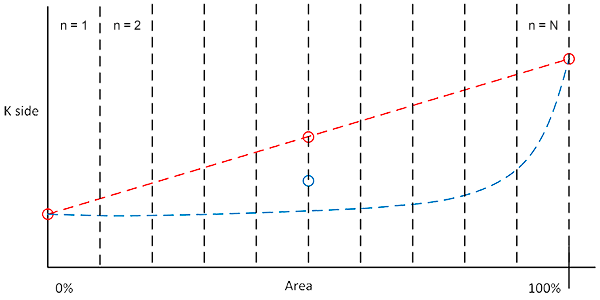# Release Notes 2.0.0.39

## Version 2.0.0.39. Build 2.0.6386.28990.

• Annular Space Heat Exchangers restrictions: Only horizontal geometries are allowed. Only sensible heat calculations are allowed. The program will raise an error when trying to calculate any condensing scenario (either in tubes or in shell) or when trying to calculate a vertical geometry for this kind of heat exchanger.
• Important changes in 'Surface Area Required' calculation algorithm:

In previous versions the side coefficients and global coefficients were calculated at the inlet and outlet of the heat exchanger. Then, the global (fouled) coefficient was taken as the average of the inlet and outlet values. The figure below shows this principle.In sensible heat calculations, for particular situations where the flow behaviour entered or passed through the transition zone, this method was not giving accurate results. The second figure illustrates this case. The red line, belonging to the old algorithm, exposes the problem. The blue line is what happens in reality for that cases: much more area is needed to work through the laminar flow stretch and the stretch for the transition zone requires much less area. The net result was that the average heat transfer coefficient calculated in previous versions of the application was too optimistic (only for these particular scenarios). The blue dot in the middle represents the real average heat transfer coefficient whereas the red dot above it, too high, was the old-mode calculated value.To be able to calculate these scenarios in a more precise way, we have changed the algorithm to a 'divide and conquer' approach: the initial whole problem is iteratively divided in 2 subproblems until the improvement gained by subdividing the problem is negligible: once that point is reached, the algorithm has converged and no further benefit is achieved (no more accurate solution is to be found) by keep on dividing the problem into smaller ones. This iterative method yields results that are far more accurate and limit the risk of under designing heat exchangers that have flow in the transition area.

Despite this improvement in the calculation, we must all understand that the transition flow zone is very irregular and not well defined. Turbulent phenomena appear but fully turbulent flow is not developed until Reynolds reaches 10000. Any heat transfer calculation in this zone has a high degree of uncertainty. If possible, always design avoiding the transition zone. If it can't be avoided, then the updated algorithm limits the risk of under design. In case of a heat exchanger found to be working in the transition zone, the program will still show a warning message.

• Other minor bug fixes and improvements.
Released: 2017-06-26
Created: 2019-12-23